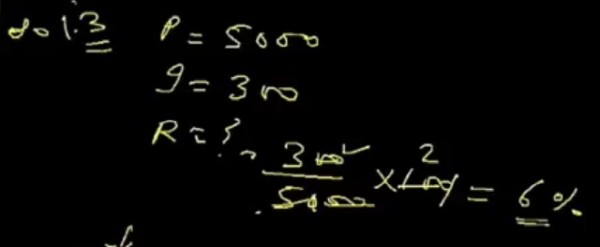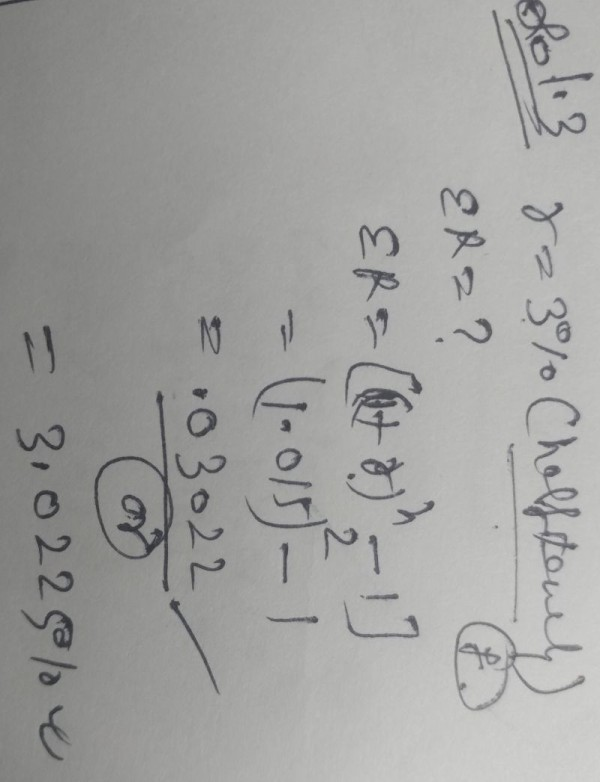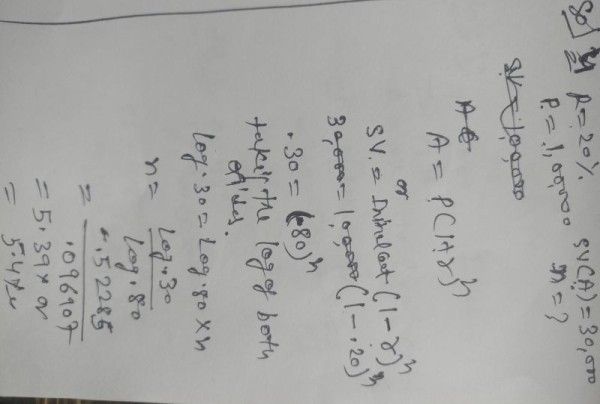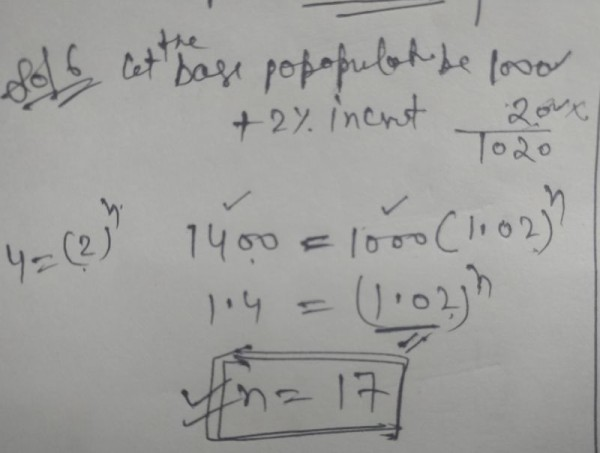CCI Online Learning
What do you want to learn today?

# Quantative aptitude problems

RishebhKumar (Studying)     17 May 2012

##### RishebhKumar
Studying
1 likes  128 points

| My Other Post

Compound interest Questions

Q1. The effective rate of interest corresponding to a normal rate 3% p.a. payable half yearly is….?

Q2. A machine is depreciated at the rate of 20% on reducing balance.The original cost of the machine was Rs.100000 and its ultimate scarp value was Rs. 30000.The effective life of machine is……..?

Q3. The population of a town increases every year by 2% of the population at the beginning of that year. The number of years by which the total increase of population be 40% is……?

Q4.The difference between C.I. and S.I. on a certain sum of money inversted for 3 years at 6% p.a. is Rs.110. The sum is….?

Q5. A machine the useful life of which is estimated to be 10 years costs Rs.10000. Rate of depreciation is 10% p.a. The scrap value at the end of its life is ….?

Q6.The effective rate of interest corresponding a nominal rate of 7% p.a. convertible quarterly is ……?

Q7. The annual birth and death rates per 1000 are 39.4 and 19.4 respectively. The number of years in which the population will be doubled assuming there is no immigration or emigration is……?

these questions are realy tough for me help guys........!!!!!!!!Tejaswi Kasturi (student-cpt)     17 May 2012

##### Tejaswi Kasturi
student-cpt
63 likes  417 points

View Profile | My Other Post

1. r = 3% p.a implies half yearly interest is 1.5 so 1+reff = 1.015^2 = 1.030225  therefore reff = 3.0225

2. new value of machine = (1-0.2)*old value i.e. 0.8*oldvalue after first year 0.8*0.8*old value is the value of the machine so ((0.8)^n)*100000 = 30000  implies (0.8)^n = 0.3  implies n = log(0.3)/log(0.8) implies n = 5.39 years

3. 1.4P = P(1.02)^n implies 1.4 = (1.02)^n taking logarithms we get n = log(1.4)/log(1.02) = 17 years(approx)

4. amount in simple interest = P + P*6*3/100 Compound interest it is P(1.06)^3 so P(1.06)^3 - P + P*6*3/100 = 110 implies P(1.191016-1.18) = 110 implies P(0.011016) = 110 implies P = 9985.48Tejaswi Kasturi (student-cpt)     17 May 2012

##### Tejaswi Kasturi
student-cpt
63 likes  417 points

View Profile | My Other Post

5.new value = (1-10/100)*old value implies new value = 0.9*(old value) so scrap value = 100000*(0.9^10 = 34867.84

6. r = 7% p.a i.e 1.75% per quarter(7/4). so 1+reff = (1.0175)^4 = 1.071859 implies reff = 7.1859

7. Let P be the population new popoulation after a year will be P*(1+birthrate)*(1-deathrate) = P*1.394*0.806 = P*1.123564

so P*(1.123564)^n = 2*P imlplies n = log(2)/log(1.123456) = 5.95 years(approx)intermediate(ipc)course (no)     17 May 2012

##### intermediate(ipc)course
no
39 likes  1460 points

View Profile | My Other Post

qt problem solution ;Brijesh Gohil   16 May 2018

##### Brijesh Gohil

2 points

View Profile | My Other Post

Give me the answer of que 2ndDKG Dexterous Zone (Institute)     01 November 2018

##### DKG Dexterous Zone
Institute
32 points

View Profile | My Other PostDKG Dexterous Zone (Institute)     01 November 2018

##### DKG Dexterous Zone
Institute
32 points

View Profile | My Other PostDKG Dexterous Zone (Institute)     01 November 2018

##### DKG Dexterous Zone
Institute
32 points

View Profile | My Other PostDKG Dexterous Zone (Institute)     01 November 2018

##### DKG Dexterous Zone
Institute
32 points

View Profile | My Other PostDKG Dexterous Zone (Institute)     01 November 2018

##### DKG Dexterous Zone
Institute
32 points

View Profile | My Other Postview more »